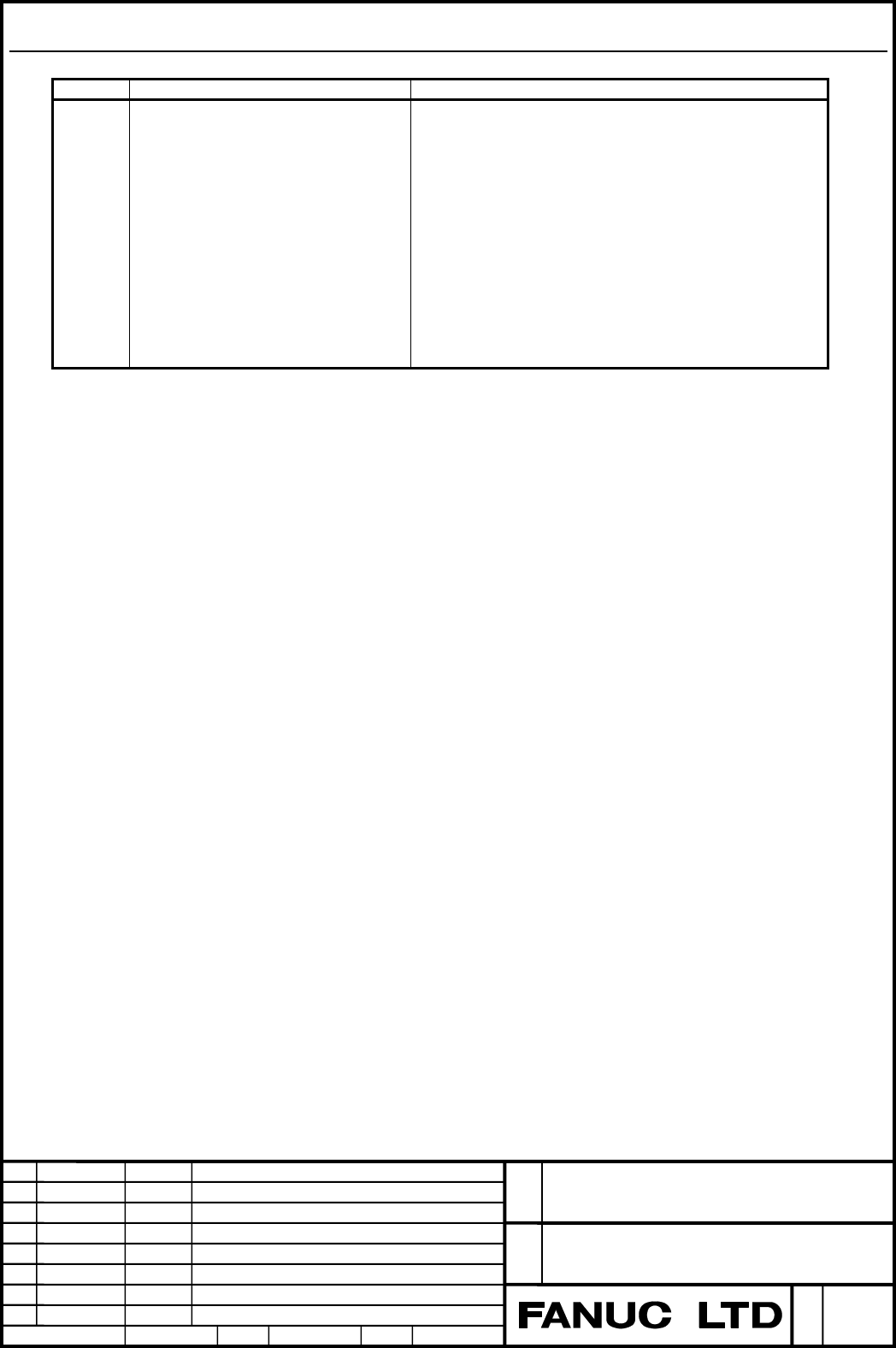# Fanuc Series 30i, Tilted Working Plane Command, The enhancement in tool axis direction command Additional Manual Page 12Edit
Apprv.
Desig.
Sheet
TITLE
Draw
No.
Date
Design
Descri
p
tion
Date
FANUC Series 30i
TILTED WORKING PLANE COMMAND
The enhancement in tool axis direction command
A
- 79449
2002.02.20 T.Sasaki
12
/
12
S.Ide
1.1.2 Alarm and message
Number Message Description
PS5459 MACHINE PARAMETER
INCORRECT
- A machine configuration parameter (parameter No.
19665 to No.19667 or 19680 to 19714) is illegal.
- The axis which is set to parameter No.19681 or
No.19686 is not a rotary axis.
- The basic three axes are not set to parameter No.1022.
- An angle does not exist within the range specified by
parameters No.19741 to No.19744 when NC calculated
the end point of rotary axes in tool center point control
for 5-axis machining (type 2) or tilted working plane
command.
- An angle does not exist when NC calculated the end
point of rotary axes in tool center point control for 5-axis
machining (type 2) or tilted working plane command.
Check the program and the machine configuration
parameters.

#### Contents Summary of Fanuc Series 30i, Tilted Working Plane Command, The enhancement in tool axis direction command Additional Manual

• Page 1FANUC Series 30i TILTED WORKING PLANE COMMAND The enhancement in tool axis direction command Specifications FANUC Series 30i TITLE TILTED WORKING PLANE COMMAND The enhancement in tool axis direction command Draw No. A- 79449 Edit Date Design Description Sheet 1/12 Date 2002.02.20 Desig. T.Sasaki App
• Page 21.1 Tilted working plane command; The enhancement in tool axis direction command Overview Generally, two rotary axes will move in tool axis direction command of tilted working plane command (G53.1). In this case, it may be desirable that the moving angle of the heavier rotary axis is smaller, or the
• Page 3When the tool axis direction command (G53.1) is specified, the "output angle" is determined form the "computed angle" as follows. If the parameter PRI(No.19608#5)=0 Tool rotation type or table rotation type machine <1> The "output angles" are represented by the computed rotary axis angle pair whose
• Page 4If the parameter PRI(No.19608#5)=1 Tool rotation type or table rotation type machine <1> The "output angles" are represented by the computed rotary axis angle pair whose slave axis (second rotary axis) moving angle is smaller. -> When the slave axis moving angle is the same -> <2> The "output angles
• Page 5CAUTION 1 If the lower limit of the movement range is larger than the upper limit, alarm PS5459 occurs when G53.1 is specified. 2 If no "computed angle" is found within the movement range because the range is too small, alarm PS5459 occurs. 3 If 0 is set for both parameters that specify the upper an
• Page 6A pair of computed angles among the pairs which are connected by the dotted line in the above figure becomes the "output angle". If the angles (the "current angles") are 50.0 (tool rotary axis) and 150.0 (table rotary axis), when G53.1 has been specified, the "output angles" are determined as follow
• Page 7(2) The case that the table moving angle is smaller and the movement range is not specified: (PRM19608#5=1, PRM19741=0, PRM19742=0, PRM19743=0, PRM19744=0) "Output angles": (Tool rotary axis 30.0, Table rotary axis 270.0) The computed angle of tool rotary axis that is nearest to the current angle is
• Page 8(3) The case that the table moving angle is smaller and the movement range is specified (i): (PRM19608#5=0, PRM19741=355.0, PRM19742=5.0, PRM19743=0, PRM19744=0) "Output angles": (Tool rotary axis 330.0, Table rotary axis 90.0) The computed angle of table rotary axis that is nearest to the current a
• Page 9(4) The case that the table moving angle is smaller and the movement range is specified (ii): (PRM19608#5=0, PRM19741=355.0, PRM19742=5.0, PRM19743=350.0, PRM19744=100.0) "Output angles": (Tool rotary axis 30.0, Table rotary axis 270.0) The computed angle of table rotary axis that is nearest to the
• Page 101.1.1 Parameters #7 #6 #5 #4 #3 #2 #1 #0 19608 PRI [Input type] Parameter input [Data type] Bit path #5 PRI Among multiple end point candidates that exist when a movement is made for a rotation axis by a command of tool center point control for 5-axis machining (type 2) or tilted working plane comma
• Page 1119742 Lower limit of the movement range of the first rotation axis [Input type] Parameter input [Data type] Real path [Unit of data] Degree [Minimum unit of data] Depend on the increment system of the reference axis [Valid data range] 9 digit of minimum unit of data (refer to standard parameter sett
• Page 121.1.2 Alarm and message Number Message Description PS5459 MACHINE PARAMETER - A machine configuration parameter (parameter No. INCORRECT 19665 to No.19667 or 19680 to 19714) is illegal. - The axis which is set to parameter No.19681 or No.19686 is not a rotary axis. - The basic three axes are not set